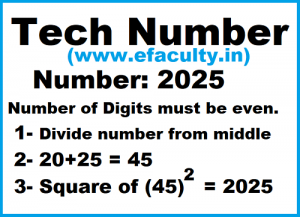# Tech Number Program in JavaA tech number can be tech number if its digits are even and the number of digits split into two number from middle then add these number if the added number’s square would be the same with the number it will called a Tech Number.

If the number is split in two equal halves,then the square of sum of these halves is equal to the number itself. Write a program to generate and print all four digits tech numbers.

Note: If number of digits is not even then it is not a tech number.

Example:

`2025 => 20+25 => 552 => 2025`
``````
import java.util.Scanner;

public class TechNumber
{
public static void main(String[] args)
{
// TODO code application logic here
int n, num, leftNumber, rightNumber, digits = 0,
sumSquare = 0;
Scanner sc = new Scanner(System.in);
System.out.print("Enter number=");
n = sc.nextInt();
num = n;
while (num > 0)
{
digits++;
num = num / 10;
}
if (digits % 2 == 0)
{
num = n;
leftNumber = num % (int) Math.pow(10, digits / 2);
rightNumber = num / (int) Math.pow(10, digits / 2);

sumSquare = (leftNumber + rightNumber) * (leftNumber + rightNumber);
if (n == sumSquare)
{
System.out.println("Tech Number");
}
else
{
System.out.println("Not Tech Number");
}
}
else
{
System.out.println("Not Tech Number");
}
}
}
``````

#### Output:

```Enter number=2025
Tech Number```
##### What is Tech Number ?
A tech number can be tech number if its digits are even and the number of digits split into two number from middle then add these number if the added number’s square would be the same with the number it will called a Tech Number. If the number is split in two equal halves,then the square of sum of these halves is equal to the number itself. Write a program to generate and print all four digits tech numbers.

##### What is Tech Number in Java?
A tech number can be tech number if its digits are even and the number of digits split into two number from middle then add these number if the added number’s square would be the same with the number it will called a Tech Number. If the number is split in two equal halves,then the square of sum of these halves is equal to the number itself. Write a program to generate and print all four digits tech numbers.

b. tech. bca icse java java tutorials learn java mca programs
Recent Posts